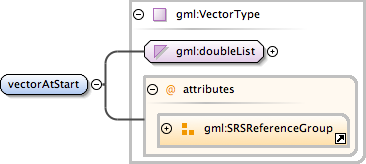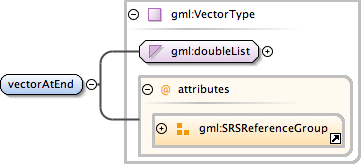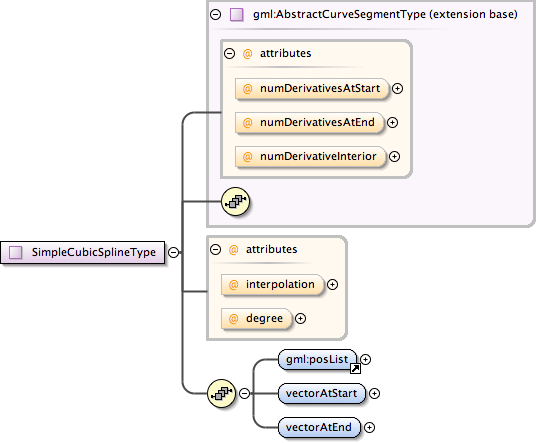### Showing:

 Annotations Attributes Diagrams Facets Properties Source Used by
Element metobj:SimpleCubicSplineType / metobj:vectorAtStart
Namespace http://xml.fmi.fi/namespace/meteorology/conceptual-model/meteorological-objects/2009/11/09
Annotations
 `"vectorAtStart" is the unit tangent vector at the start point of the spline.`
DiagramType gml:VectorType
Type hierarchy
Properties
 content: complex
Attributes
QName Type Fixed Default Use Annotation
axisLabels gml:NCNameList optional
 ```Ordered list of labels for all the axes of this CRS. The gml:axisAbbrev value should be used for these axis labels, after spaces and forbiddden characters are removed. When the srsName attribute is included, this attribute is optional. When the srsName attribute is omitted, this attribute shall also be omitted.```
srsDimension positiveInteger optional
 ```The "srsDimension" is the length of coordinate sequence (the number of entries in the list). This dimension is specified by the coordinate reference system. When the srsName attribute is omitted, this attribute shall be omitted.```
srsName anyURI optional
 ```In general this reference points to a CRS instance of gml:CoordinateReferenceSystemType (see coordinateReferenceSystems.xsd). For well known references it is not required that the CRS description exists at the location the URI points to. If no srsName attribute is given, the CRS must be specified as part of the larger context this geometry element is part of, e.g. a geometric element like point, curve, etc. It is expected that this attribute will be specified at the direct position level only in rare cases.```
uomLabels gml:NCNameList optional
 ```Ordered list of unit of measure (uom) labels for all the axes of this CRS. The value of the string in the gml:catalogSymbol should be used for this uom labels, after spaces and forbiddden characters are removed. When the axisLabels attribute is included, this attribute shall also be included. When the axisLabels attribute is omitted, this attribute shall also be omitted.```
Source
 ``` "vectorAtStart" is the unit tangent vector at the start point of the spline. ```
Schema location http://xml.fmi.fi/schema/meteorology/conceptual-model/meteorological-objects/2009/11/09/metobjects-common-geometry.xsd
Element metobj:SimpleCubicSplineType / metobj:vectorAtEnd
Namespace http://xml.fmi.fi/namespace/meteorology/conceptual-model/meteorological-objects/2009/11/09
Annotations
 `"vectorAtEnd" is the unit tangent vector at the end point of the spline.`
DiagramType gml:VectorType
Type hierarchy
Properties
 content: complex
Attributes
QName Type Fixed Default Use Annotation
axisLabels gml:NCNameList optional
 ```Ordered list of labels for all the axes of this CRS. The gml:axisAbbrev value should be used for these axis labels, after spaces and forbiddden characters are removed. When the srsName attribute is included, this attribute is optional. When the srsName attribute is omitted, this attribute shall also be omitted.```
srsDimension positiveInteger optional
 ```The "srsDimension" is the length of coordinate sequence (the number of entries in the list). This dimension is specified by the coordinate reference system. When the srsName attribute is omitted, this attribute shall be omitted.```
srsName anyURI optional
 ```In general this reference points to a CRS instance of gml:CoordinateReferenceSystemType (see coordinateReferenceSystems.xsd). For well known references it is not required that the CRS description exists at the location the URI points to. If no srsName attribute is given, the CRS must be specified as part of the larger context this geometry element is part of, e.g. a geometric element like point, curve, etc. It is expected that this attribute will be specified at the direct position level only in rare cases.```
uomLabels gml:NCNameList optional
 ```Ordered list of unit of measure (uom) labels for all the axes of this CRS. The value of the string in the gml:catalogSymbol should be used for this uom labels, after spaces and forbiddden characters are removed. When the axisLabels attribute is included, this attribute shall also be included. When the axisLabels attribute is omitted, this attribute shall also be omitted.```
Source
 ``` "vectorAtEnd" is the unit tangent vector at the end point of the spline. ```
Schema location http://xml.fmi.fi/schema/meteorology/conceptual-model/meteorological-objects/2009/11/09/metobjects-common-geometry.xsd
Complex Type metobj:SimpleCubicSplineType
Namespace http://xml.fmi.fi/namespace/meteorology/conceptual-model/meteorological-objects/2009/11/09
Annotations
 ```Cubic splines are similar to line strings in that they are a sequence of segments each with its own defining function. A cubic spline uses the control points and a set of derivative parameters to define a piecewise 3rd degree polynomial interpolation. Unlike line-strings, the parameterization by arc length is not necessarily still a polynomial. The function describing the curve must be C2, that is, have a continuous 1st and 2nd derivative at all points, and pass through the controlPoints in the order given. Between the control points, the curve segment is defined by a cubic polynomial. At each control point, the polynomial changes in such a manner that the 1st and 2nd derivative vectors are the same from either side. The control parameters record must contain vectorAtStart, and vectorAtEnd which are the unit tangent vectors at controlPoint and controlPoint[n] where n = controlPoint.count. Note: only the direction of the vectors is relevant, not their length.```
DiagramType extension of gml:AbstractCurveSegmentType
Type hierarchy
Used by
 Element metobj:SimpleCubicSpline
Model gml:posList , metobj:vectorAtStart , metobj:vectorAtEnd
Children gml:posList, metobj:vectorAtEnd, metobj:vectorAtStart
Attributes
QName Type Fixed Default Use Annotation
degree integer 3 optional
 `The degree for a cubic spline is "3".`
interpolation gml:CurveInterpolationType cubicSpline optional
 ```The attribute "interpolation" specifies the curve interpolation mechanism used for this segment. This mechanism uses the control points and control parameters to determine the position of this curve segment. For a CubicSpline the interpolation is fixed as "cubicSpline".```
numDerivativeInterior integer 0 optional
 ```The attribute "numDerivativesInterior" specifies the type of continuity that is guaranteed interior to the curve. The default value of "0" means simple continuity, which is a mandatory minimum level of continuity. This level is referred to as "C 0 " in mathematical texts. A value of 1 means that the function and its first derivative are continuous at the appropriate end point: "C 1 " continuity. A value of "n" for any integer means the function and its first n derivatives are continuous: "C n " continuity. NOTE: Use of these values is only appropriate when the basic curve definition is an underdetermined system. For example, line string segments cannot support continuity above C 0 , since there is no spare control parameter to adjust the incoming angle at the end points of the segment. Spline functions on the other hand often have extra degrees of freedom on end segments that allow them to adjust the values of the derivatives to support C 1 or higher continuity.```
numDerivativesAtEnd integer 0 optional
 ```The attribute "numDerivativesAtEnd" specifies the type of continuity between this curve segment and its successor. If this is the last curve segment in the curve, one of these values, as appropriate, is ignored. The default value of "0" means simple continuity, which is a mandatory minimum level of continuity. This level is referred to as "C 0 " in mathematical texts. A value of 1 means that the function and its first derivative are continuous at the appropriate end point: "C 1 " continuity. A value of "n" for any integer means the function and its first n derivatives are continuous: "C n " continuity. NOTE: Use of these values is only appropriate when the basic curve definition is an underdetermined system. For example, line string segments cannot support continuity above C 0 , since there is no spare control parameter to adjust the incoming angle at the end points of the segment. Spline functions on the other hand often have extra degrees of freedom on end segments that allow them to adjust the values of the derivatives to support C 1 or higher continuity.```
numDerivativesAtStart integer 0 optional
 ```The attribute "numDerivativesAtStart" specifies the type of continuity between this curve segment and its predecessor. If this is the first curve segment in the curve, one of these values, as appropriate, is ignored. The default value of "0" means simple continuity, which is a mandatory minimum level of continuity. This level is referred to as "C 0 " in mathematical texts. A value of 1 means that the function and its first derivative are continuous at the appropriate end point: "C 1 " continuity. A value of "n" for any integer means the function and its first n derivatives are continuous: "C n " continuity. NOTE: Use of these values is only appropriate when the basic curve definition is an underdetermined system. For example, line string segments cannot support continuity above C 0 , since there is no spare control parameter to adjust the incoming angle at the end points of the segment. Spline functions on the other hand often have extra degrees of freedom on end segments that allow them to adjust the values of the derivatives to support C 1 or higher continuity.```
Source
 ``` Cubic splines are similar to line strings in that they are a sequence of segments each with its own defining function. A cubic spline uses the control points and a set of derivative parameters to define a piecewise 3rd degree polynomial interpolation. Unlike line-strings, the parameterization by arc length is not necessarily still a polynomial. The function describing the curve must be C2, that is, have a continuous 1st and 2nd derivative at all points, and pass through the controlPoints in the order given. Between the control points, the curve segment is defined by a cubic polynomial. At each control point, the polynomial changes in such a manner that the 1st and 2nd derivative vectors are the same from either side. The control parameters record must contain vectorAtStart, and vectorAtEnd which are the unit tangent vectors at controlPoint and controlPoint[n] where n = controlPoint.count. Note: only the direction of the vectors is relevant, not their length. Unlike in gml:CubicSplineType, positions can only be specified in one way: inlined using gml:posList. The number of direct positions in the list must be at least three. "vectorAtStart" is the unit tangent vector at the start point of the spline. "vectorAtEnd" is the unit tangent vector at the end point of the spline. The attribute "interpolation" specifies the curve interpolation mechanism used for this segment. This mechanism uses the control points and control parameters to determine the position of this curve segment. For a CubicSpline the interpolation is fixed as "cubicSpline". The degree for a cubic spline is "3". ```
Schema location http://xml.fmi.fi/schema/meteorology/conceptual-model/meteorological-objects/2009/11/09/metobjects-common-geometry.xsd
Attribute metobj:SimpleCubicSplineType / @interpolation
Namespace No namespace
Annotations
 ```The attribute "interpolation" specifies the curve interpolation mechanism used for this segment. This mechanism uses the control points and control parameters to determine the position of this curve segment. For a CubicSpline the interpolation is fixed as "cubicSpline".```
Type gml:CurveInterpolationType
Properties
 fixed: cubicSpline
Facets
 enumeration linear enumeration geodesic enumeration circularArc3Points enumeration circularArc2PointWithBulge enumeration circularArcCenterPointWithRadius enumeration elliptical enumeration clothoid enumeration conic enumeration polynomialSpline enumeration cubicSpline enumeration rationalSpline
Used by
 Complex Type metobj:SimpleCubicSplineType
Source
 ``` The attribute "interpolation" specifies the curve interpolation mechanism used for this segment. This mechanism uses the control points and control parameters to determine the position of this curve segment. For a CubicSpline the interpolation is fixed as "cubicSpline". ```
Schema location http://xml.fmi.fi/schema/meteorology/conceptual-model/meteorological-objects/2009/11/09/metobjects-common-geometry.xsd
Attribute metobj:SimpleCubicSplineType / @degree
Namespace No namespace
Annotations
 `The degree for a cubic spline is "3".`
Type integer
Properties
 fixed: 3
Used by
 Complex Type metobj:SimpleCubicSplineType
Source
 ``` The degree for a cubic spline is "3". ```
Schema location http://xml.fmi.fi/schema/meteorology/conceptual-model/meteorological-objects/2009/11/09/metobjects-common-geometry.xsd